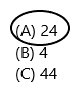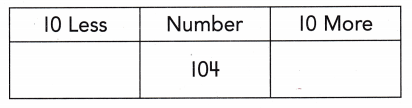# Texas Go Math Grade 1 Lesson 10.7 Answer Key 10 More, 10 Less

Refer to our Texas Go Math Grade 1 Answer Key Pdf to score good marks in the exams. Test yourself by practicing the problems from Texas Go Math Grade 1 Lesson 10.7 Answer Key 10 More, 10 Less.

## Texas Go Math Grade 1 Lesson 10.7 Answer Key 10 More, 10 Less

Essential Question
How can you identify numbers that are 10 more or 10 less than a number?
10 more or 10 less gives strategies for mentally finding less than a two-digit number without having to count. This strategy is done by adding or subtracting a one from the ten’s place.
For example:
To find 10 more than 25, you add 1 to the 2 to get 35.
To find 10 less than 25, you subtract 1 from the 2 to get 15.
This method is key for developing mental maths skills.

Explore
Useto solve. Draw quick pictures to show your work.For The Teacher

• Read the following problem. Tony has 2 boxes of markers and 2 more markers. Pat has 10 fewer markers than Tony. Jan has 10 more markers than Tony. How many markers does each child have?
Pat has 2 markers.
Jan has 22 markers.
Explanation:
The number of markers tony has=10+2=12.
The number of markers Pat have=12-10=2 (because pat is having 10 less than tony so I subtracted )
The number of markers Jan is having=12+10=22 (because Jan is having 10 more than tony so I added).Math Talk
Mathematical Processes
What number has less 10 than 12? Explain.
Explanation:
10 more or 10 less gives strategies for mentally finding less than a two-digit number without having to count. This strategy is done by adding or subtracting a one from the ten’s place.
Here asked the number 10 less than 12.
12-10=2.
We need to subtract 10 from 12 then we get the answer.

Model and Draw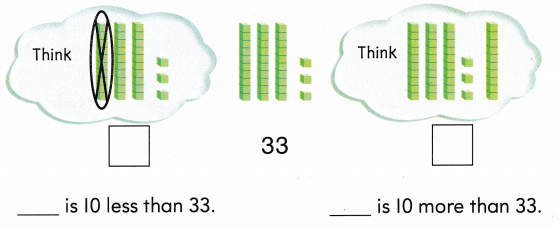Explanation:
10 more or 10 less gives strategies for mentally finding less than a two-digit number without having to count. This strategy is done by adding or subtracting a one from the ten’s place.Share and Show

Use mental math. Write the numbers that are 10 less and 10 more.

Question 1.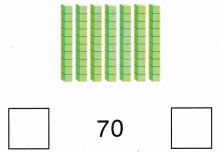10 more: 70+10=80
Explanation:
10 more or 10 less gives strategies for mentally finding less than a two-digit number without having to count. This strategy is done by adding or subtracting a one from the ten’s place.Question 2.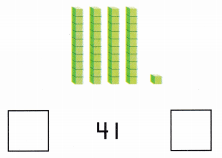10 more: 41+10=51
Explanation:
10 more or 10 less gives strategies for mentally finding less than a two-digit number without having to count. This strategy is done by adding or subtracting a one from the ten’s place.Question 3.10 more: 58+10=68
Explanation:
10 more or 10 less gives strategies for mentally finding less than a two-digit number without having to count. This strategy is done by adding or subtracting a one from the ten’s place.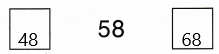Question 4.10 more: 66+10=76
Explanation:
10 more or 10 less gives strategies for mentally finding less than a two-digit number without having to count. This strategy is done by adding or subtracting a one from the ten’s place.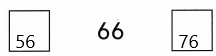Question 5.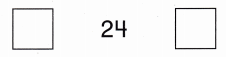10 more: 24+10=34
Explanation:
10 more or 10 less gives strategies for mentally finding less than a two-digit number without having to count. This strategy is done by adding or subtracting a one from the ten’s place.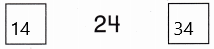Question 6.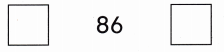10 more: 86+10=96
Explanation:
10 more or 10 less gives strategies for mentally finding less than a two-digit number without having to count. This strategy is done by adding or subtracting a one from the ten’s place.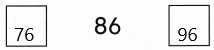Question 7.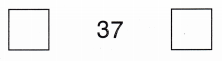10 more: 37+10=47
Explanation:
10 more or 10 less gives strategies for mentally finding less than a two-digit number without having to count. This strategy is done by adding or subtracting a one from the ten’s place.Question 8.10 more: 15+10=25
Explanation:
10 more or 10 less gives strategies for mentally finding less than a two-digit number without having to count. This strategy is done by adding or subtracting a one from the ten’s place.Problem Solving

Use mental math. Complete the chart.

Question 9.10 more: 39+10=49
Explanation:
10 more or 10 less gives strategies for mentally finding less than a two-digit number without having to count. This strategy is done by adding or subtracting a one from the ten’s place.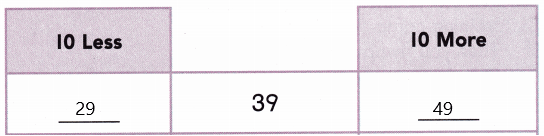Question 10.10 more: 75+10=85
Explanation:
10 more or 10 less gives strategies for mentally finding less than a two-digit number without having to count. This strategy is done by adding or subtracting a one from the ten’s place.Question 11.10 more: 90+10=100
Explanation:
10 more or 10 less gives strategies for mentally finding less than a two-digit number without having to count. This strategy is done by adding or subtracting a one from the ten’s place.Question 12.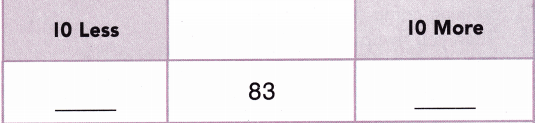83+10=93
Explanation:
10 more or 10 less gives strategies for mentally finding less than a two-digit number without having to count. This strategy is done by adding or subtracting a one from the ten’s place.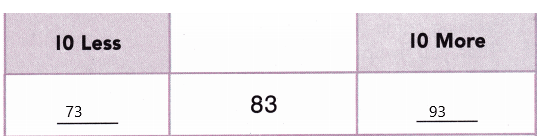Question 13.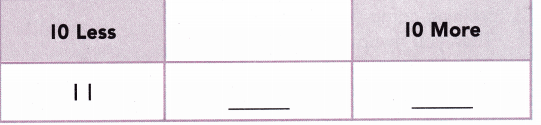10 more of 21=31
Explanation:
10 more or 10 less gives strategies for mentally finding less than a two-digit number without having to count. This strategy is done by adding or subtracting a one from the ten’s place.
21-10=11
21+10=31.Question 14.
H.O.T. Solve. I have 89 rocks. I want to collect 10 more. How many rocks will I have then?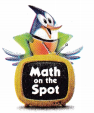___ rocks
Explanation:
The number of rocks I have=89
The number of rocks I need to collect=10
The total number of rocks after collecting 10 more=89+10=99.
Therefore, I have 99 rocks then.

Question 15.
H.O.T. Multi-Step Margo has 28 stamps. Chet has 10 more stamps than Margo. Luis has 10 more stamps than Chet. How many stamps does Luis have?Explanation:
The number of stamps Margo has=28
The number of stamps Chet has more than Margo=10.
The total number of stamps Chet has=28+10=38
The number of stamps Luis have more than Chet=10
The total number of stamps Luis has=38+10=48.
Therefore, Luis has 48 stamps.

Question 16.
You can practice flying at a superhero training camp. You fly up 86 feet. Then you fly up 10 more feet. How far are you above the ground?
(A) 106 feet
(B) 96 feet
(C) 76 feetExplanation:
The number of feet the flying practice=86
The number of feet they increase more=10
The total number of feet=86+10=96
Therefore, the total feet is 96.

Question 7.
Display Look at the chart. What are the unknown numbers?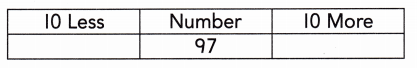(A) 87 and 107
(B) 96 and 98
(C) 107 and 117Explanation:
10 more or 10 less gives strategies for mentally finding 10 more or less than a two-digit number without having to count. This strategy is done by adding or subtracting a one from the ten’s place.

Question 19.
Texas Test Prep What number is 10 less than 76?
(A) 66
(B) 86
(C) 75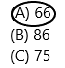10 less: 76-10=66
Explanation:
10 less gives strategies for mentally finding less than a two-digit number without having to count. This strategy is done by adding or subtracting a one from the ten’s place.

Take Home Activity

• Write a two digit number. such as 25, 40, or 81, Ask your child to identify the numbers that are ten less than and ten more than that number. Repeat with other numbers.
25: 10 more: 25+10=35
10 less: 25-10=15
40: 10 more: 40+10=50
10 less: 40-10=30
81: 10 more: 81+10=91
10 less: 81-10=71

### Texas Go Math Grade 1 Lesson 10.7 Homework and Practice Answer Key

Use mental math. Write the numbers that are 10 less and 10 more.

Question 1.10 more: 40+10=50
10 less: 40-10=30Explanation:
10 more or 10 less gives strategies for mentally finding 10 more or less than a two-digit number without having to count. This strategy is done by adding or subtracting a one from the ten’s place.

Question 2.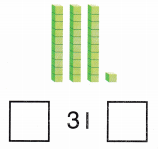10 more: 31+10=41
10 less: 31-10=21Explanation:
10 more or 10 less gives strategies for mentally finding 10 more or less than a two-digit number without having to count. This strategy is done by adding or subtracting a one from the ten’s place.
Question 3.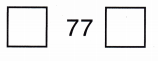10 more: 77+10=88
10 less: 77-10=66Explanation:
10 more or 10 less gives strategies for mentally finding 10 more or less than a two-digit number without having to count. This strategy is done by adding or subtracting a one from the ten’s place.

Question 4.10 more: 13+10=23
10 less: 13-10=3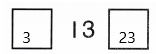Explanation:
10 more or 10 less gives strategies for mentally finding 10 more or less than a two-digit number without having to count. This strategy is done by adding or subtracting a one from the ten’s place.

Question 5.
A bird lands on a branch of a tree. The branch is 33 feet above the ground. Then the bird flies to a branch that is 10 feet higher. How far ¡s the bird above the ground?
___ feet
Explanation:
The number of feet above the ground=33
The number of feet the birds flies higher=10
The total number of feet =33+10=43.
Therefore, the bird flies above the ground is 43.

Texas Test Prep

Lesson Check

Question 6.
A group of hikers camp 82 feet up on a mountain. The next night they camp 10 feet higher. How far up the mountain did they camp?(A) 92 feet
(B) 72 feet
(C) 102 feet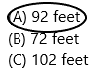Explanation:
The number of feet they hike on mountain=82
The number of feet they hike on next night=10
The total number of feet they hike on mountains=82+10=92.
Therefore, the group members hike on mountains are 92 feet.

Question 7.
Multi-Step Carl has 87 toy cars. He gives 10 cars to Elena. Then Mark gives Carl 10 cars. How many cars does Carl have now?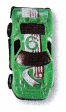(A) 77
(B) 67
(C) 87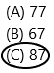Explanation:
The number of car toys Carl has=87
The number of cars Carl gave to Elena=10
Now the total number of cars Carl have=87-10=77
The number of cars Mark gave to Carl=10
Again Carl got 10 cars more because Mark gave 10 car toys to Carl.
The total number of cars Carl have now=77+10=87.

Question 8.
What number is 10 less than 34?
(A) 24
(B) 4
(C) 44